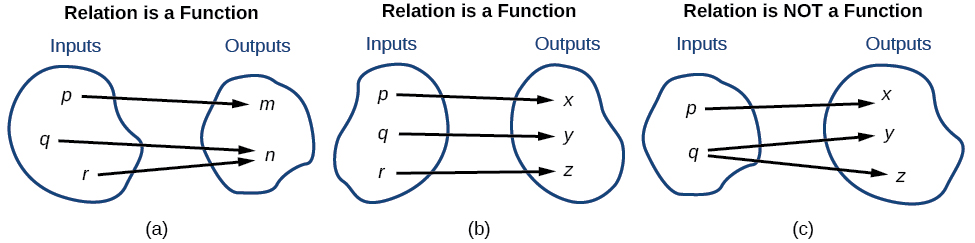# 3.1 Functions and function notation

 Page 1 / 21
In this section, you will:
• Determine whether a relation represents a function.
• Find the value of a function.
• Determine whether a function is one-to-one.
• Use the vertical line test to identify functions.
• Graph the functions listed in the library of functions.

A jetliner changes altitude as its distance from the starting point of a flight increases. The weight of a growing child increases with time. In each case, one quantity depends on another. There is a relationship between the two quantities that we can describe, analyze, and use to make predictions. In this section, we will analyze such relationships.

## Determining whether a relation represents a function

A relation    is a set of ordered pairs. The set consisting of the first components of each ordered pair    is called the domain and the set consisting of the second components of each ordered pair is called the range . Consider the following set of ordered pairs. The first numbers in each pair are the first five natural numbers. The second number in each pair is twice that of the first.

$\left\{\left(1,\text{\hspace{0.17em}}2\right),\text{\hspace{0.17em}}\left(2,\text{\hspace{0.17em}}4\right),\text{\hspace{0.17em}}\left(3,\text{\hspace{0.17em}}6\right),\text{\hspace{0.17em}}\left(4,\text{\hspace{0.17em}}8\right),\text{\hspace{0.17em}}\left(5,\text{\hspace{0.17em}}10\right)\right\}$

The domain is $\left\{1,\text{\hspace{0.17em}}2,\text{\hspace{0.17em}}3,\text{\hspace{0.17em}}4,\text{\hspace{0.17em}}5\right\}.$ The range is $\left\{2,\text{\hspace{0.17em}}4,\text{\hspace{0.17em}}6,\text{\hspace{0.17em}}8,\text{\hspace{0.17em}}10\right\}.$

Note that each value in the domain is also known as an input value, or independent variable    , and is often labeled with the lowercase letter $\text{\hspace{0.17em}}x.\text{\hspace{0.17em}}$ Each value in the range is also known as an output value, or dependent variable    , and is often labeled lowercase letter $\text{\hspace{0.17em}}y.$

A function $\text{\hspace{0.17em}}f\text{\hspace{0.17em}}$ is a relation that assigns a single element in the range to each element in the domain . In other words, no x -values are repeated. For our example that relates the first five natural numbers    to numbers double their values, this relation is a function because each element in the domain, $\left\{1,\text{\hspace{0.17em}}2,\text{\hspace{0.17em}}3,\text{\hspace{0.17em}}4,\text{\hspace{0.17em}}5\right\},$ is paired with exactly one element in the range, $\left\{2,\text{\hspace{0.17em}}4,\text{\hspace{0.17em}}6,\text{\hspace{0.17em}}8,\text{\hspace{0.17em}}10\right\}.$

Now let’s consider the set of ordered pairs that relates the terms “even” and “odd” to the first five natural numbers. It would appear as

$\left\{\left(\text{odd},\text{\hspace{0.17em}}1\right),\text{\hspace{0.17em}}\left(\text{even},\text{\hspace{0.17em}}2\right),\text{\hspace{0.17em}}\left(\text{odd},\text{\hspace{0.17em}}3\right),\text{\hspace{0.17em}}\left(\text{even},\text{\hspace{0.17em}}4\right),\text{\hspace{0.17em}}\left(\text{odd},\text{\hspace{0.17em}}5\right)\right\}$

Notice that each element in the domain, $\left\{\text{even,}\text{\hspace{0.17em}}\text{odd}\right\}$ is not paired with exactly one element in the range, $\left\{1,\text{\hspace{0.17em}}2,\text{\hspace{0.17em}}3,\text{\hspace{0.17em}}4,\text{\hspace{0.17em}}5\right\}.$ For example, the term “odd” corresponds to three values from the domain, $\left\{1,\text{\hspace{0.17em}}3,\text{\hspace{0.17em}}5\right\}$ and the term “even” corresponds to two values from the range, $\left\{2,\text{\hspace{0.17em}}4\right\}.$ This violates the definition of a function, so this relation is not a function.

[link] compares relations that are functions and not functions.(a) This relationship is a function because each input is associated with a single output. Note that input   q   and   r   both give output   n .   (b) This relationship is also a function. In this case, each input is associated with a single output. (c) This relationship is not a function because input     q   is associated with two different outputs.

## Function

A function    is a relation in which each possible input value leads to exactly one output value. We say “the output is a function of the input.”

The input    values make up the domain    , and the output    values make up the range    .

Given a relationship between two quantities, determine whether the relationship is a function.

1. Identify the input values.
2. Identify the output values.
3. If each input value leads to only one output value, classify the relationship as a function. If any input value leads to two or more outputs, do not classify the relationship as a function.

answer and questions in exercise 11.2 sums
what is a algebra
what is the identity of 1-cos²5x equal to?
__john __05
Kishu
Hi
Abdel
hi
Ye
hi
Nokwanda
C'est comment
Abdel
Hi
Amanda
hello
SORIE
Hiiii
Chinni
hello
Ranjay
hi
ANSHU
hiiii
Chinni
h r u friends
Chinni
yes
Hassan
so is their any Genius in mathematics here let chat guys and get to know each other's
SORIE
I speak French
Abdel
okay no problem since we gather here and get to know each other
SORIE
hi im stupid at math and just wanna join here
Yaona
lol nahhh none of us here are stupid it's just that we have Fast, Medium, and slow learner bro but we all going to work things out together
SORIE
it's 12
what is the function of sine with respect of cosine , graphically
tangent bruh
Steve
cosx.cos2x.cos4x.cos8x
sinx sin2x is linearly dependent
what is a reciprocal
The reciprocal of a number is 1 divided by a number. eg the reciprocal of 10 is 1/10 which is 0.1
Shemmy
Reciprocal is a pair of numbers that, when multiplied together, equal to 1. Example; the reciprocal of 3 is ⅓, because 3 multiplied by ⅓ is equal to 1
Jeza
each term in a sequence below is five times the previous term what is the eighth term in the sequence
I don't understand how radicals works pls
How look for the general solution of a trig function
stock therom F=(x2+y2) i-2xy J jaha x=a y=o y=b
sinx sin2x is linearly dependent
cr
root under 3-root under 2 by 5 y square
The sum of the first n terms of a certain series is 2^n-1, Show that , this series is Geometric and Find the formula of the n^th
cosA\1+sinA=secA-tanA
Wrong question
why two x + seven is equal to nineteen.
The numbers cannot be combined with the x
Othman
2x + 7 =19
humberto
2x +7=19. 2x=19 - 7 2x=12 x=6
Yvonne
because x is 6
SAIDIByBy Yasser IbrahimBy OpenStaxBy Rohini AjayByBy OpenStaxBy Hoy WenBy Janet ForresterBy Rylee MinllicBy Karen GowdeyBy